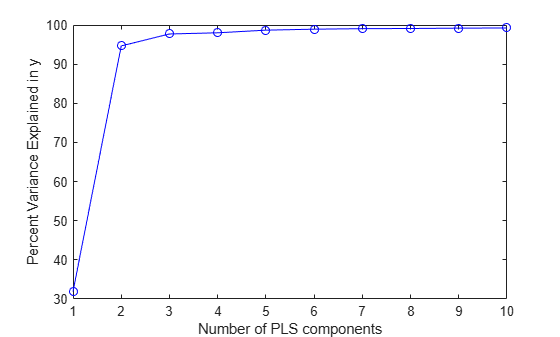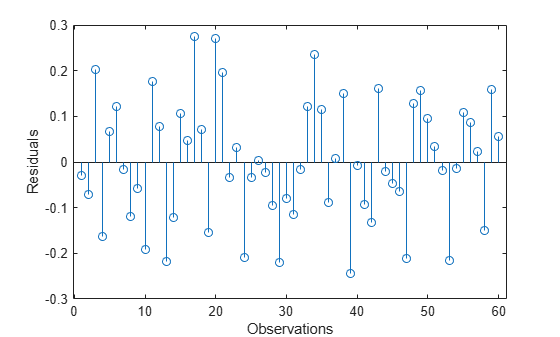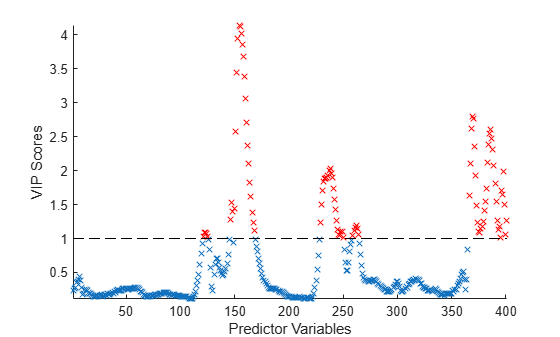# plsregress

Partial least-squares (PLS) regression

## Syntax

``[XL,YL] = plsregress(X,Y,ncomp)``
``[XL,YL,XS,YS,BETA,PCTVAR,MSE,stats] = plsregress(X,Y,ncomp)``
``[XL,YL,XS,YS,BETA,PCTVAR,MSE,stats] = plsregress(___,Name,Value)``

## Description

````[XL,YL] = plsregress(X,Y,ncomp)` returns the predictor and response loadings `XL` and `YL`, respectively, for a partial least-squares (PLS) regression of the responses in matrix `Y` on the predictors in matrix `X`, using `ncomp` PLS components.```

example

````[XL,YL,XS,YS,BETA,PCTVAR,MSE,stats] = plsregress(X,Y,ncomp)` also returns: The predictor scores `XS`. Predictor scores are PLS components that are linear combinations of the variables in `X`.The response scores `YS`. Response scores are linear combinations of the responses with which the PLS components `XS` have maximum covariance.A matrix `BETA` of coefficient estimates for PLS regression. `plsregress` adds a column of ones in the matrix `X` to compute coefficient estimates for a model with constant terms (intercept).The percentage of variance `PCTVAR` explained by the regression model.The estimated mean squared errors `MSE` for PLS models with `ncomp` components.A structure `stats` that contains the PLS weights, T2 statistic, and predictor and response residuals. ```
````[XL,YL,XS,YS,BETA,PCTVAR,MSE,stats] = plsregress(___,Name,Value)` specifies options using one or more name-value arguments in addition to any of the input argument combinations in previous syntaxes. The name-value arguments specify `MSE` calculation parameters. For example, `'cv',5` calculates the `MSE` using 5-fold cross-validation.```

## Examples

collapse all

Load the `spectra` data set. Create the predictor `X` as a numeric matrix that contains the near infrared (NIR) spectral intensities of 60 samples of gasoline at 401 wavelengths. Create the response `y` as a numeric vector that contains the corresponding octane ratings.

```load spectra X = NIR; y = octane;```

Perform PLS regression with 10 components of the responses in `y` on the predictors in `X`.

`[XL,yl,XS,YS,beta,PCTVAR] = plsregress(X,y,10);`

Plot the percent of variance explained in the response variable (`PCTVAR`) as a function of the number of components.

```plot(1:10,cumsum(100*PCTVAR(2,:)),'-bo'); xlabel('Number of PLS components'); ylabel('Percent Variance Explained in y');```Compute the fitted response and display the residuals.

```yfit = [ones(size(X,1),1) X]*beta; residuals = y - yfit; stem(residuals) xlabel('Observations'); ylabel('Residuals');```Calculate variable importance in projection (VIP) scores for a partial least-squares (PLS) regression model. You can use VIP to select predictor variables when multicollinearity exists among variables. Variables with a VIP score greater than 1 are considered important for the projection of the PLS regression model .

Load the `spectra` data set. Create the predictor `X` as a numeric matrix that contains the near infrared (NIR) spectral intensities of 60 samples of gasoline at 401 wavelengths. Create the response `y` as a numeric vector that contains the corresponding octane ratings. Specify the number of components `ncomp`.

```load spectra X = NIR; y = octane; ncomp = 10;```

Perform PLS regression with 10 components of the responses in y on the predictors in `X`.

`[XL,yl,XS,YS,beta,PCTVAR,MSE,stats] = plsregress(X,y,ncomp);`

Calculate the normalized PLS weights.

`W0 = stats.W ./ sqrt(sum(stats.W.^2,1));`

Calculate the VIP scores for `ncomp` components.

```p = size(XL,1); sumSq = sum(XS.^2,1).*sum(yl.^2,1); vipScore = sqrt(p* sum(sumSq.*(W0.^2),2) ./ sum(sumSq,2));```

Find variables with a VIP score greater than or equal to 1.

`indVIP = find(vipScore >= 1);`

Plot the VIP scores.

```scatter(1:length(vipScore),vipScore,'x') hold on scatter(indVIP,vipScore(indVIP),'rx') plot([1 length(vipScore)],[1 1],'--k') hold off axis tight xlabel('Predictor Variables') ylabel('VIP Scores')```## Input Arguments

collapse all

Predictor variables, specified as a numeric matrix. `X` is an n-by-p matrix, where n is the number of observations and p is the number of predictor variables. Each row of `X` represents one observation, and each column represents one variable. `X` must have the same number of rows as `Y`.

Data Types: `single` | `double`

Response variables, specified as a numeric matrix. `Y` is an n-by-m matrix, where n is the number of observations and m is the number of response variables. Each row of `Y` represents one observation, and each column represents one variable. Each row in `Y` is the response for the corresponding row in `X`.

Data Types: `single` | `double`

Number of components, specified as a numeric vector. If you do not specify `ncomp`, the default value is ```min(size(X,1) – 1,size(X,2))```.

Data Types: `single` | `double`

### Name-Value Pair Arguments

Specify optional comma-separated pairs of `Name,Value` arguments. `Name` is the argument name and `Value` is the corresponding value. `Name` must appear inside quotes. You can specify several name and value pair arguments in any order as `Name1,Value1,...,NameN,ValueN`.

Example: `'cv',10,'Options',statset('UseParallel',true)` calculates the `MSE` using 10-fold cross-validation, where computations run in parallel.

`MSE` calculation method, specified as `'resubstitution'`, a positive integer, or a `cvpartition` object.

• Specify `'cv'` as `'resubstitution'` to use both `X` and `Y` to fit the model and estimate the mean squared errors, without cross-validation.

• Specify `'cv'` as a positive integer `k` to use `k`-fold cross-validation.

• Specify `'cv'` as a `cvpartition` object to specify another type of cross-validation partition.

Example: `'cv',5`

Example: `'cv',cvpartition(n,'Holdout',0.3)`

Data Types: `single` | `double` | `char` | `string`

Number of Monte Carlo repetitions for cross-validation, specified as a positive integer. If you specify `'cv'` as `'resubstitution'`, then `'mcreps'` must be 1.

Example: `'mcreps',5`

Data Types: `single` | `double`

Options for running computations in parallel and setting random streams, specified as a structure. Create the `Options` structure with `statset`. This table lists the option fields and their values.

Field NameValueDefault
`UseParallel`Set this value to `true` to run computations in parallel.`false`
`UseSubstreams`

Set this value to `true` to run computations in parallel in a reproducible manner.

To compute reproducibly, set `Streams` to a type that allows substreams: `'mlfg6331_64'` or `'mrg32k3a'`.

`false`
`Streams`Specify this value as a `RandStream` object or a cell array consisting of one such object.If you do not specify `Streams`, then `plsregress` uses the default stream.

Note

You need Parallel Computing Toolbox™ to run computations in parallel.

Example: `'Options',statset('UseParallel',true)`

Data Types: `struct`

## Output Arguments

collapse all

Predictor loadings, returned as a numeric matrix. `XL` is a p-by-`ncomp` matrix, where p is the number of predictor variables and `ncomp` is the number of PLS components. Each row of `XL` contains coefficients that define a linear combination of PLS components approximating the original predictor variables.

Data Types: `single` | `double`

Response loadings, returned as a numeric matrix. `YL` is an m-by-`ncomp` matrix, where m is the number of response variables and `ncomp` is the number of PLS components. Each row of `YL` contains coefficients that define a linear combination of PLS components approximating the original response variables.

Data Types: `single` | `double`

Predictor scores, returned as a numeric matrix. `XS` is an n-by-`ncomp` orthonormal matrix, where n is the number of observations and `ncomp` is the number of PLS components. Each row of `XS` corresponds to one observation, and each column corresponds to one component.

Data Types: `single` | `double`

Response scores, returned as a numeric matrix. `YS` is an n-by-`ncomp` matrix, where n is the number of observations and `ncomp` is the number of PLS components. Each row of `YS` corresponds to one observation, and each column corresponds to one component. `YS` is not orthogonal or normalized.

Data Types: `single` | `double`

Coefficient estimates for PLS regression, returned as a numeric matrix. `BETA` is a (p + 1)-by-m matrix, where p is the number of predictor variables and m is the number of response variables. The first row of `BETA` contains coefficient estimates for the constant terms.

Data Types: `single` | `double`

Percentage of variance explained by the model, returned as a numeric matrix. `PCTVAR` is a 2-by-`ncomp` matrix, where `ncomp` is the number of PLS components. The first row of `PCTVAR` contains the percentage of variance explained in `X` by each PLS component, and the second row contains the percentage of variance explained in `Y`.

Data Types: `single` | `double`

Mean squared error, returned as a numeric matrix. `MSE` is a 2-by-(`ncomp` + 1) matrix, where `ncomp` is the number of PLS components. `MSE` contains the estimated mean squared errors for a PLS model with `ncomp` components. The first row of `MSE` contains mean squared errors for the predictor variables in `X`, and the second row contains mean squared errors for the response variables in `Y`. The column j of `MSE` contains mean squared errors for j – 1 components.

Data Types: `single` | `double`

Model statistics, returned as a structure with the fields described in this table.

FieldDescription
`W`p-by-`ncomp` matrix of PLS weights so that `XS = X0*W`
`T2`T2 statistic for each point in `XS`
`Xresiduals`Predictor residuals, ```X0 – XS*XL'```
`Yresiduals`Response residuals, ```Y0 – XS*YL'```

For more information about the centered predictor and response variables `X0` and `Y0`, see Algorithms.

## Algorithms

`plsregress` uses the SIMPLS algorithm . The function first centers `X` and `Y` by subtracting the column means to get the centered predictor and response variables `X0` and `Y0`, respectively. However, the function does not rescale the columns. To perform PLS regression with standardized variables, use `zscore` to normalize `X` and `Y` (columns of `X0` and `Y0` are centered to have mean 0 and scaled to have standard deviation 1).

After centering `X` and `Y`, `plsregress` computes the singular value decomposition (SVD) on `X0'*Y0`. The predictor and response loadings `XL` and `YL` are the coefficients obtained from regressing `X0` and `Y0` on the predictor score `XS`. You can reconstruct the centered data `X0` and `Y0` using `XS*XL'` and `XS*YL'`, respectively.

`plsregress` initially computes `YS` as `YS = Y0*YL`. By convention , however, `plsregress` then orthogonalizes each column of `YS` with respect to preceding columns of `XS`, so that `XS'*YS` is a lower triangular matrix.

 de Jong, Sijmen. “SIMPLS: An Alternative Approach to Partial Least Squares Regression.” Chemometrics and Intelligent Laboratory Systems 18, no. 3 (March 1993): 251–63. https://doi.org/10.1016/0169-7439(93)85002-X.

 Rosipal, Roman, and Nicole Kramer. "Overview and Recent Advances in Partial Least Squares." Subspace, Latent Structure and Feature Selection: Statistical and Optimization Perspectives Workshop (SLSFS 2005), Revised Selected Papers (Lecture Notes in Computer Science 3940). Berlin, Germany: Springer-Verlag, 2006, vol. 3940, pp. 34–51. https://doi.org/10.1007/11752790_2.

 Chong, Il-Gyo, and Chi-Hyuck Jun. “Performance of Some Variable Selection Methods When Multicollinearity Is Present.” Chemometrics and Intelligent Laboratory Systems 78, no. 1–2 (July 2005) 103–12. https://doi.org/10.1016/j.chemolab.2004.12.011.